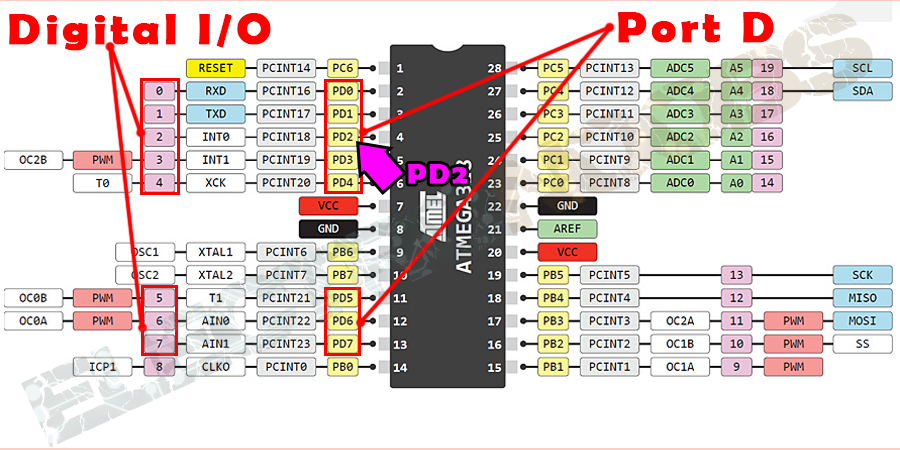Arduino

# Arduino Interrupts | Improve your Programming SkillsJanuary 31, 2020 by Utsource Parts
ShareIn this article, I will give an introduction to interrupts and how to use them in an easy way!

## Hardware

 1 Arduino Uno 1 Button
``````const int buttonPin = 2;     // the number of the pushbutton pin
const int ledPin =  13;      // the number of the LED pin

// variables will change:
volatile int buttonState = 0;         // variable for reading the pushbutton status

void setup() {
// initialize the LED pin as an output:
pinMode(ledPin, OUTPUT);
// initialize the pushbutton pin as an input:
pinMode(buttonPin, INPUT);
// Attach an interrupt to the ISR vector
attachInterrupt(0, pin_ISR, CHANGE);
}

void loop() {
// Nothing here!
}

void pin_ISR() {
digitalWrite(ledPin, buttonState);
}``````

In this article, I will give an introduction to interrupts and how to use them in an easy way!

What are Interrupts?

Interrupts are, literally Interrupts!!

Interruption in real life is really similar concept to what is used in Arduino and other Microcontrollers.

There can be different types of interrupts, for example, if someone gives input high or low on a pin or timer interrupts, which do something when a particular amount of time has passed.

So, to keep things easy and simple, we will be only using Arduino Uno or atmega328 because the interrupt handling and execution are different for other microcontrollers.

Following is the simple way you can use interrupts.

## Interrupts with a Button:

First, let us have a look at simple default button sketch from Arduino IDE->Examples->Basics->Button

``````const int buttonPin = 2;     // the number of the pushbutton pin
const int ledPin =  13;      // the number of the LED pin

// variables will change:
int buttonState = 0;         // variable for reading the pushbutton status

void setup() {
// initialize the LED pin as an output:
pinMode(ledPin, OUTPUT);
// initialize the pushbutton pin as an input:
pinMode(buttonPin, INPUT);
}

void loop() {
// read the state of the pushbutton value:

// check if the pushbutton is pressed.
// if it is, the buttonState is HIGH:
if (buttonState == HIGH) {
// turn LED on:
digitalWrite(ledPin, HIGH);
}
else {
// turn LED off:
digitalWrite(ledPin, LOW);
}
}``````

The above code example runs a continuous loop in "void loop ()" section to check if the button is pressed.

This way of checking whether the button is pressed is really simple but not really what you want to do when you have quite a ling piece of code running on the Arduino, for example, if your program takes for example 0.5 Second to run one loop, and the same button code from examples is in the bottom, the program will only check whether the button is pressed only after the intervals of 0.5 Seconds, and every button press which happens during the time of rest of program execution will be ignored.

Now to solve the above mentioned problem, we need to "Interrupt " the microcontroller from whatever it is doing to do what we want it to, this in a nutshell is what known as interrupt handeling, and the things or the routine that you want the microcontroller to follow after getting interrupt, is known as Interrupt Service Routine or ISP in short.

Following code does the same thing as the above one but uses interrupt to check if the button is pressed.

``````const int buttonPin = 2;     // the number of the pushbutton pin
const int ledPin =  13;      // the number of the LED pin

// variables will change:
volatile int buttonState = 0;         // variable for reading the pushbutton status

void setup() {
// initialize the LED pin as an output:
pinMode(ledPin, OUTPUT);
// initialize the pushbutton pin as an input:
pinMode(buttonPin, INPUT);
// Attach an interrupt to the ISR vector
attachInterrupt(0, pin_ISR, CHANGE);
}

void loop() {
// Nothing here!
}

void pin_ISR() {
digitalWrite(ledPin, buttonState);
}``````

Now, let us dissect the code to know what it is doing and how.

A Volatile Variable is declared at the beginning to store the state of the button:

``volatile int buttonState = 0;         // variable for reading the pushbutton status``

Now the real magic begins in the void setup () part, the new thing here is:

``````// Attach an interrupt to the ISR vector
attachInterrupt(0, pin_ISR, CHANGE);``````

This attachInterrupt() function takes three parameters:

attachInterrupt(Pin, ISR, Mode)

The pin is the pin you want to attach the interrupt to, in our case we have taken the pin 0 as the interrupt.

ISR is the Interrupt Service Routine, this is the function under which we define what should be done after getting the interrupt.

Mode defines when the interrupt should be triggered. Four constants are predefined as valid values:

LOW to trigger the interrupt whenever the pin is low,

CHANGE to trigger the interrupt whenever the pin changes the value

RISING to trigger when the pin goes from low to high,

FALLING for when the pin goes from high to low.

Now, the function void pin_ISR() is the function under which we define what to do whatever you want to do whenever a particular interrupt is detected. We simply use the digitalWrite() function.

``````void pin_ISR() {
digitalWrite(ledPin, buttonState);
}``````